Math Central - mathcentral.uregina.caResource Room— glossaries Resource RoomMathematics Glossary - Middle Years
Maxine Stinka, Saskatchewan Education, Curriculum and Instruction

Note: The definitions included here are those that are used in the Saskatchewan Education document "Mathematics 6-9: A Curriculum Guide for the Middle Level".

Various mathematics dictionaries may have different definitions. These definitions are designed to be meaningful to middle level mathematics teachers.

Select the first letter of the term that you wish to look for.
A | B | C | D | E | F | H | I | J | K | L | M | N | O | P | Q | R | S | T | U | V | W | X | Y | Z

P

parallelogram

• a quadrilateral with both pairs of opposite sides parallel.

percent

• a ratio where the second term is 100.

perfect square

• a whole number that is the square of an integer. e.g. 25 is a perfect square because 5 X 5 = 25.

perpendicular

• two segments or lines that intersect to form right angles.

pi

plane of symmetry

• a plane that divides a 3-D object into 2 parts, each a mirror image of the other.

polygon

• a closed plane figure bounded by line segments.

polyhedron

• a 3-D object that has polygons as its faces. The intersection of any two faces forms an edge.
•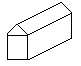polynomial

• an expression consisting of one or more terms, including some variable(s).
E.g. 3 x2 - 2 x + 1.

population

• in statistics, population refers to the entire group about which data are being collected.

power

primary data

• data obtained directly using methods such as a survey or an experiment.

prime number

• an integer greater than 1 whose only positive factors are itself and one.

prism

• a geometric solid with two bases that are congruent, parallel polygons and all other faces are parallelograms. It is referred to as a right prism if the faces are rectangles. A prisms is named according to the shape of its bases. e.g. triangular prism
•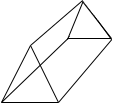proper fraction

• a fraction whose numerator is less than its denominator.

proportion

• an equality of two ratios. e.g. 5 : 8 = 10 : 16.

protractor (circular)

• a circular device subdivided into 360 equal parts and used for measuring angles.

protractor (semi-circular)

• a half circle subdivided around the edge in degrees and used for measuring angles.

Pythagorean Theorem

• in any right triangle, the square of the length of the hypotenuse equals the sum of the squares of the lengths of the other 2 sides.
•  c2 = a2 + b2.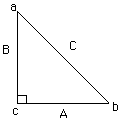pyramid

• a geometric solid with one base that is a polygon and all other faces are triangles with a common vertex.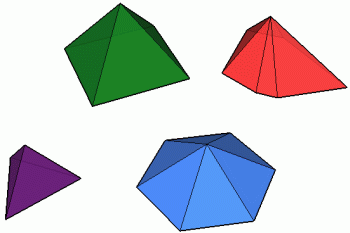Q

• when the axes are drawn in a coordinate plane, the plane is divided into 4 sections called quadrants.
•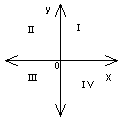• a polygon with four sides.
quartile

• any one of the values in a frequency distribuion that divides the distribution into four parts of equal frequency. The first quartile is the number below which 1/4 of the values are found.about math central :: site map :: links :: notre site français* Registered trade mark of Imperial Oil Limited. Used under license.